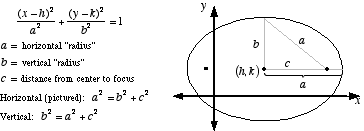index: click on a letter A B C D E F G H I J K L M N O P Q R S T U V W X Y Z A to Z index index: subject areas numbers & symbols sets, logic, proofs geometry algebra trigonometry advanced algebra & pre-calculus calculus advanced topics probability & statistics real world applications multimedia entrieswww.mathwords.com about mathwords website feedback

 Ellipse A conic section which is essentially a stretched circle. Formally, an ellipse can be defined as follows: For two given points, the foci, an ellipse is the locus of points such that the sum of the distance to each focus is constant. The standard form for the equation of an ellipse is given below.See also